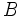Difference between revisions of "Pareto efficiency criterion"

• Because of this problem of Pareto efficiency being too weak to yield unique allocations, we often consider a modified version where gainers are in a position to compensate losers (known as the compensation principle). For instance, an outcome in which$A$ gets 100 units and B gets 3 units is not a Pareto improvement over an outcome in which$A$ and$B$ both get 5 units, but if we allow$A$ to provide partial compensation to$B$, we can get an outcome that is a Pareto improvement over an outcome where$A$ and$B$ both get 5 units. A modification of the Pareto criterion that accounts for potential compensation (Without necessarily including the compensation as part of the arrangement itself) is termed the Kaldor-Hicks efficiency criterion, also called the potential Pareto criterion.